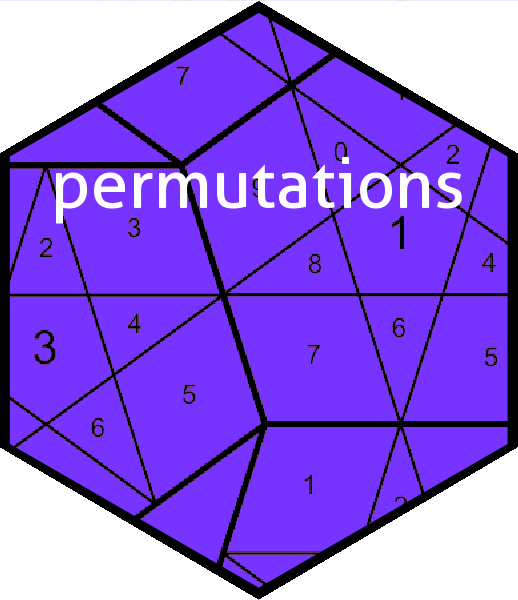This vignette discusses the concept of cyclists as used in the permutations package.

A “cyclist” is an object corresponding to a permutation P. It is a list with elements that are integer vectors corresponding to the cycles of P. This object is informally known as a cyclist, but there is no S3 class corresponding to it. For example

list(c(1,3,4), c(8,9), c(2,5))
## []
##  1 3 4
##
## []
##  8 9
##
## []
##  2 5

is a cyclist of three cycles: $$(134)$$, $$(89)$$, and $$(25)$$. It corresponds to the permutation $$(134)(89)(25)$$, or $$\left(\begin{array}{ccccccccc}1&2&3&4&5&6&7&8&9\\9&2&6&3&5&4&1&7&8\end{array}\right)$$.

An object of S3 class cycle is a (possibly named) list of cyclists. NB: there is an unavoidable notational clash here. When considering a single permutation, “cycle” means group-theoretic cycle; when considering R objects, “cycle” means an R object of class cycle whose elements are permutations written in cycle form. The elements of a cyclist are the disjoint group-theoretic cycles; in the example above, the group-theoretic cycles were $$(134)$$, $$(89)$$, and $$(25)$$.

A cyclist may be poorly formed in a number of ways: the cycles may include repeats, or contain elements which are common to more than one cycle. Such problems are detected by cyclist_valid():

cyclist_valid(list(c(1,-3,4), c(8,9), c(2,5)))
## Warning in cyclist_valid(list(c(1, -3, 4), c(8, 9), c(2, 5))): negative
## elements
##  FALSE
cyclist_valid(list(c(0,3,4), c(8,9), c(2,5)))
## Warning in cyclist_valid(list(c(0, 3, 4), c(8, 9), c(2, 5))): zero element
##  FALSE
cyclist_valid(list(c(1.2,3,4), c(8,9), c(2,5)))
## Warning in cyclist_valid(list(c(1.2, 3, 4), c(8, 9), c(2, 5))): non-integer
## entries
##  FALSE
cyclist_valid(list(c(3,3,4), c(8,9), c(2,5)))
## Warning in cyclist_valid(list(c(3, 3, 4), c(8, 9), c(2, 5))): repeated value
##  FALSE
cyclist_valid(list(c(1,3,4), c(1,9), c(2,5)))
## Warning in cyclist_valid(list(c(1, 3, 4), c(1, 9), c(2, 5))): repeated value
##  FALSE

Even a well-formed cyclist possesses numerous redundancies: firstly, because the cycles commute, their order is immaterial (the problem is, in R a list is ordered. It might have been better to represent a cyclist as a set of (group theoretic) cycles). Secondly, the cycles themselves are invariant under cyclic permutation: cycle (567) is identical to (675) and (756). In the package, we need cyclists to have a standardised form. To this end, function nicify_cyclist() takes a cyclist and puts it in a nice form but does not alter the permutation. It takes a cyclist and orders each cycle so that the smallest element appears first (that is, it changes (523) to (235)). It then orders the cycles by the smallest element:

a <- list(c(9,3,4), c(8,1), c(2,5))
a
## []
##  9 3 4
##
## []
##  8 1
##
## []
##  2 5
nicify_cyclist(a)
## []
##  1 8
##
## []
##  2 5
##
## []
##  3 4 9

Also, there are less serious problems: the cycles may include length-one cycles; the cycles may start with an element that is not the smallest (these are not serious in the sense that their presence does not destroy the interpretation of the argument as a permutation). These issues are dealt with by nicify_cyclist(), which calls helper function remove_length_one():

a <- list(c(9,3,4), 7, c(8,1), c(2,5))
a
## []
##  9 3 4
##
## []
##  7
##
## []
##  8 1
##
## []
##  2 5
nicify_cyclist(a)
## []
##  1 8
##
## []
##  2 5
##
## []
##  3 4 9

Function nicify_cyclist() is called automatically in the package by cycle() so we are guaranteed that cycle objects are nicified. The package includes functionality to convert between word and cycle form and the low-level helper function is vec2cyclist_single(). This takes a vector of integers, interpreted as a word, and converts it into a cyclist. Length-one cycles are discarded:

v <- c(1,2,4,3,5,9,6,7,8)
v
##  1 2 4 3 5 9 6 7 8
as.word(v)
##  (34)(6987)
## [coerced from word form]
vec2cyclist_single(v)
## []
##  3 4
##
## []
##  6 9 8 7

This function is potentially a bottleneck and one of my long-term ideas is to write it in C. Function vec2cyclist_single_cpp() is placeholder function that is not yet written.

Function cyclist2word_single() takes a cyclist and returns a vector corresponding to a single word. This function is not intended for everyday use; function cycle2word() is much more user-friendly.

cyclist2word_single(list(c(1,3,4),c(8,7,9)))
##  3 2 4 1 5 6 9 7 8

The package includes some rudimentary functionality to coerce strings to cycles. Function char2cyclist_single() takes a character string and returns a cyclist:

char2cyclist_single("(3)(139)")
## []
##  3
##
## []
##  1 3 9
char2cyclist_single("(342)(19)")
## []
##  3 4 2
##
## []
##  1 9

Above, note that the first call does not give a well-specified cyclist (the number 3 is repeated). Function char2cyclist_single() does not return an error. To catch this kind of problem use the more user-friendly function char2cycle(), which calls the validity checks. This function returns a cyclist which is not necessarily canonicalized: it might have length-one cycles, and the cycles themselves might start with the wrong number. The function attempts to deal with absence of commas in a sensible way, so (18,19)(2,5) is dealt with appropriately too. The function is insensitive to spaces.

The user-friendly version char2cycle() performs canonicalization and also checks for malformed cyclists.

## Package idiom

Most users will not deal with cyclists directly. Function cycle() takes a list of cyclists and returns an object of class cycle. It nicifies its input before returning it:

(a <- list(list(c(1,2,4),c(3,6)),list(c(1,2),c(3,4,5,6,7))))
## []
## [][]
##  1 2 4
##
## [][]
##  3 6
##
##
## []
## [][]
##  1 2
##
## [][]
##  3 4 5 6 7
cycle(a)
##  (124)(36)   (12)(34567)

However, it might be more convenient in practice to use as.cycle():

as.cycle(c("(124)(36)", "(12)(34567)"))
##  (124)(36)   (12)(34567)